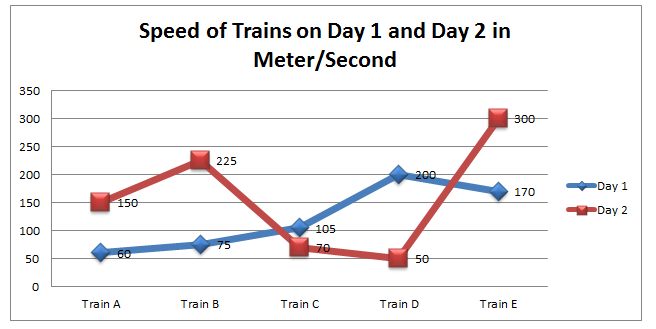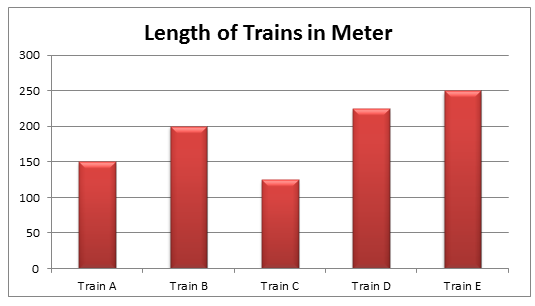# Double Diagram Data Interpretation Set: Part 5

Directions (1 to 5): Following Line Graph represents the uniform speed of Trains (A, B, C, D and E) on two different days in meter per second and the Bar Chart represents the length of each train in meter.1. Approximately what is the average time taken by train D on day 1 and day 2 to cross the platform of length 500 meter?

A. 6 Sec
B. 9 Sec
C. 12 Sec
D. Data Insufficient
E. None of these

2. At what speed must train C travel on Day 2 such that it crosses the platform of 500 meter in half of the expected time?
A. 139 m/s
B.149 m/s
C. 159 m/s
D. Data insufficient
E. None of these

3. What is the average speed of all the trains on Day 1 in Km/Hr?
A. 449
B. 459
C. 429
D. Data insufficient
E. None of these

4. What will be the total time travelled by train B, C & D on Day 1?
A. 554 minutes
B. 224 minutes
C. 334 minutes
D. Data insufficient
E. None of these

5. What is the ratio of Percentage increase or decrease in speed of Train A from day 1 to day 2 to Train D from day 1 to day 2?
A. 4:1
B. 3:1
C. 2:1
D. Data insufficient
E. None of these

#### 1. Option B

Time= Distance/Speed
Time taken on day 1, t1= (train length + Platform Length)/speed
= (225+500)/200
= 3.6 sec
Time taken on day 2, t2= (train length + Platform Length)/speed
= (225+500)/50
= 14.5 sec
Average time = (t1+t2)/2
= 9 seconds (ans)

#### 2. Option A

Expected time = (125+500)/ 70
= 9 seconds
Speed needed to cross the platform in 4.5 sec = (125+ 500)/4.5
= 139 m/s (ans)

#### 3. Option E

Average speed on day 1 = total speed/5
= (60+75+105+200+170)/5
= 610/5
= 122 m/s
Average speed on day 1 in km/hr = 122*(18/5)
= 439 km/hr (ans)

#### 4. Option D

Without knowing the distance travelled time cannot be calculated, so data is not sufficient.

#### 5. Option C

% increase in speed of Train A= [(150-60)/60]*100
= 150 %
% decrease in speed of Train D= [(200-50)/200]*100
= 75 %
Ratio = 150: 75
= 2: 1(ans)